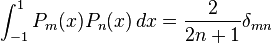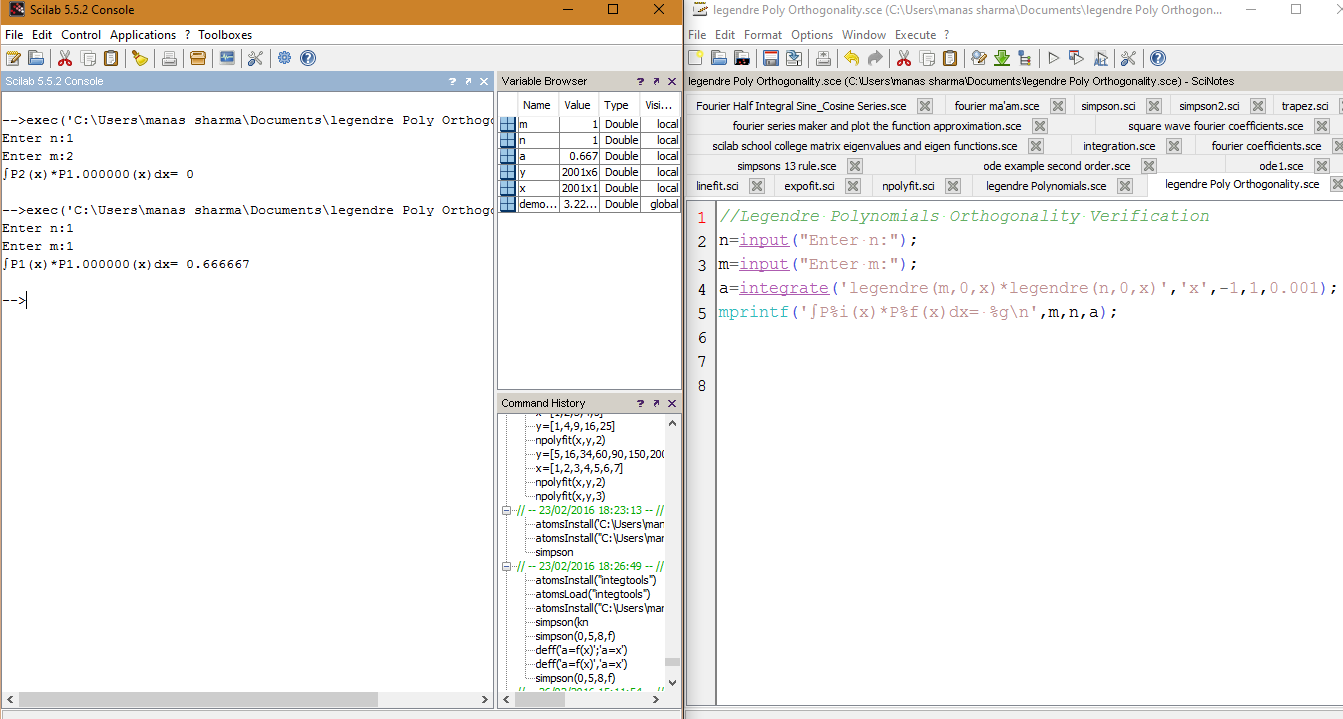# Checking the Orthogonality of Legendre Functions through SCILAB

Legendre functions have an important property that is, they are orthogonal on the interval −1 ≤ x ≤ 1:(where δmn denotes the Kronecker delta, equal to 1 if m = n and to 0 otherwise).

We can verify this result using Scilab.

To work with Legendre Polynomials we use the Scilab function legendre(n,m,x).

Which basically returns the value of the Associated Legendre Polynomial for a given value of m,n and x.
However, since I only wanted Legendre Polynomials so I’ll have to put m=0.

The following code returns the value of the integral, ∫Pm(x)*Pn(x)dx,

```//Legendre Polynomials Orthogonality Verification
clc;
n=input("Enter n:");
m=input("Enter m:");
a=integrate('legendre(m,0,x)*legendre(n,0,x)','x',-1,1,0.001);
mprintf('∫P%i(x)*P%f(x)dx= %g\n',m,n,a);
```

Output:
Enter n:1
Enter m:1
∫P1(x)*P1.000000(x)dx= 0.666667

Enter n:5
Enter m:2
∫P2(x)*P5.000000(x)dx= 0[wpedon id="7041" align="center"]